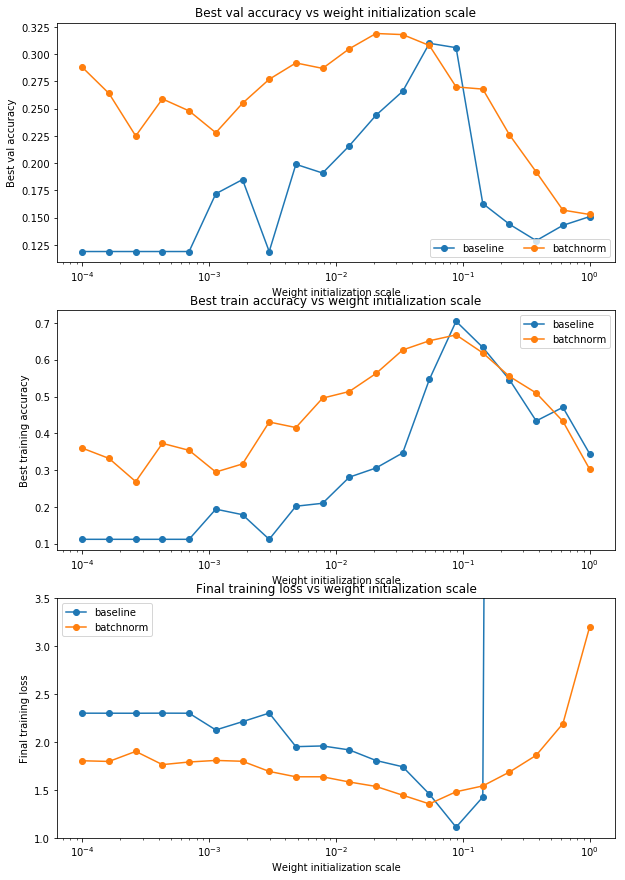# [cs231n] assignment2 Batch Nomalization部分

BN操作的主要公式如下：

$$\mu = \frac{1}{m} \sum_{1}^{m}x_i$$

$$\sigma = \sum_{i=1}^{m}(x_i-\mu)^2$$

$$\widehat{x} = \frac{x_i – \mu} {\sqrt{\sigma ^ 2+ \epsilon}}$$

$$y_i = \gamma \widehat{x_i} + \beta$$BN层的主要作用如下：

BN解决了反向传播过程中的梯度问题（梯度消失和爆炸），同时使得不同scale的 w 整体更新步调更一致。在神经网络训练时遇到收敛速度很慢，或梯度爆炸等无法训练的状况时可以尝试BN来解决。另外，在一般使用情况下也可以加入BN来加快训练速度，提高模型精度。
* 减少坏初始化的影响；
* 加快模型的收敛速度；
* 可以用大些的学习率
* 能有效地防止过拟合。